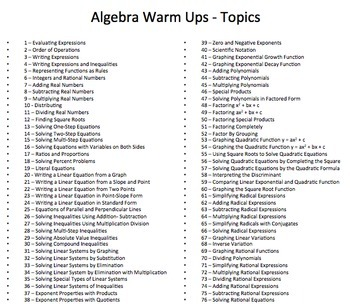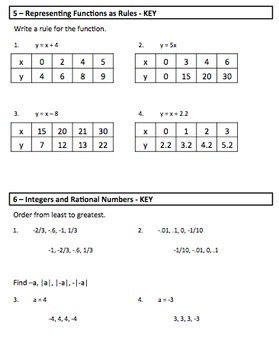# Algebra Warm UpsSubject
Resource Type
Format
PDF (7 MB|79 pages)
Standards
\$12.00
\$12.00

### Description

Algebra Warm Ups

Great for a "Back to School Algebra Review" or
"Summer Algebra Review" too!

Perfect as a review over the summer. With just 4 questions a day for 76 days, students will be fully prepared for the new school year!!!

Products you may also like...

You may wish to use my popular Math Foldables with this product found here.

Algebra Daily Math Warm Ups with Key

I have created daily warm ups with questions from 76 specific Algebra topics. I use these warm ups for my Algebra I, Algebra II and even Geometry students. There are over 300 questions included. Please download my free preview. An answer key is provided.

Two warm ups are on each page to save paper.

Visit my store for 7th and 8th grade math warm ups that are aligned to the common core and other algebra resources.

Topics - 4 questions per topic

1 – Evaluating Expressions
2 – Order of Operations
3 – Writing Expressions
4 – Writing Expressions and Inequalities
5 – Representing Functions as Rules
6 – Integers and Rational Numbers
8 – Subtracting Real Numbers
9 – Multiplying Real Numbers
10 - Distributing
11 – Dividing Real Numbers
12 – Finding Square Roots
13 – Solving One-Step Equations
14 – Solving Two-Step Equations
15 – Solving Multi-Step Equations
16 – Solving Equations with Variables on Both Sides
17 – Ratios and Proportions
18 – Solving Percent Problems
19 – Literal Equations
20 - Writing a Linear Equation from a Graph
21 – Writing a Linear Equation from a Slope and Point
22 – Writing a Linear Equation from Two Points
23 – Writing a Linear Equation in Point-Slope Form
24 – Writing a Linear Equation in Standard Form
25 – Equations of Parallel and Perpendicular Lines
26 – Solving Inequalities Using Addition- Subtraction
27 – Solving Inequalities Using Multiplication Division
28 – Solving Multi-Step Inequalities
29 – Solving Absolute Value Inequalities
30 – Solving Compound Inequalities
31 – Solving Linear Systems by Graphing
32 – Solving Linear Systems by Substitution
33 – Solving Linear Systems by Elimination
34 – Solving Linear System by Elimination with Multiplication
35 – Solving Special Types of Linear Systems
36 – Solving Linear Systems of Inequalities
37 – Exponent Properties with Products
38 – Exponent Properties with Quotients
39 – Zero and Negative Exponents
40 – Scientific Notation
41 – Graphing Exponential Growth Function
42 – Graphing Exponential Decay Function
44 – Subtracting Polynomials
45 – Multiplying Polynomials
46 – Special Products
47 – Solving Polynomials in Factored Form
48 – Factoring x2 + bx + c
49 – Factoring ax2 + bx + c
50 – Factoring Special Products
51 – Factoring Completely
52 – Factor By Grouping
53 – Graphing Quadratic Function y = ax2 + c
54 – Graphing the Quadratic Function y = ax2 + bx + c
55 – Using Square Roots to Solve Quadratic Equations
56 – Solving Quadratic Equations by Completing the Square
58 – Interpreting the Discriminant
59 – Comparing Linear Exponential and Quadratic Function
60 – Graphing the Square Root Function
65 – Simplifying Radicals with Conjugates
67 – Graphing Linear Variations
68 – Inverse Variation
69 – Graphing Rational Functions
70 – Dividing Polynomials
71 – Simplifying Rational Expressions
72 – Multiplying Rational Expressions
73 – Dividing Rational Expressions
75 – Subtracting Rational Expressions
76 – Solving Rational Equations

Connect with Me

Math to the Core on Facebook
Math to the Core Blog
Math to the Core on Pinterest
Math to the Core on Twitter
Math to the Core on Instagram

Total Pages
79 pages
Included
Teaching Duration
N/A
Report this Resource to TpT
Reported resources will be reviewed by our team. Report this resource to let us know if this resource violates TpT’s content guidelines.

### Standards

to see state-specific standards (only available in the US).
Interpret the parameters in a linear or exponential function in terms of a context.
For exponential models, express as a logarithm the solution to 𝘢𝘣 to the 𝘤𝘵 power = 𝘥 where 𝘢, 𝘤, and 𝘥 are numbers and the base 𝘣 is 2, 10, or 𝘦; evaluate the logarithm using technology.
Observe using graphs and tables that a quantity increasing exponentially eventually exceeds a quantity increasing linearly, quadratically, or (more generally) as a polynomial function.
Construct linear and exponential functions, including arithmetic and geometric sequences, given a graph, a description of a relationship, or two input-output pairs (include reading these from a table).
Understand the inverse relationship between exponents and logarithms and use this relationship to solve problems involving logarithms and exponents.